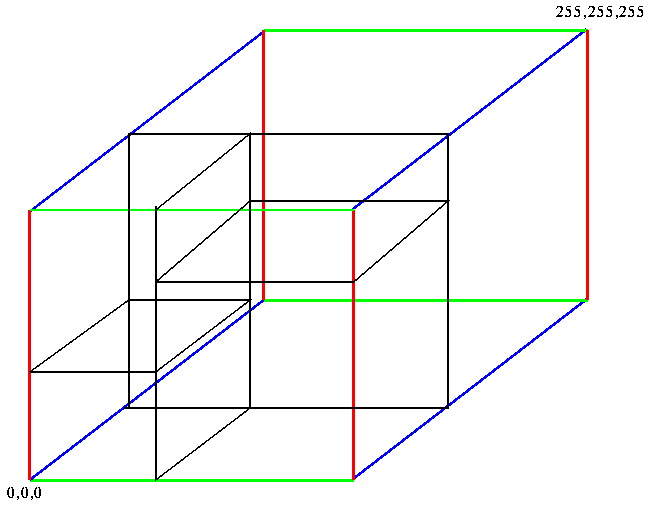## Median Cut Algorithms

The premise behind median cut algorithms is to have every entry in the color map represent the same number of pixels in the original image. In contrast to uniform sub-division, these algorithms divide the color space based on the distribution of the original colors. The process is as follows:
1. Find the smallest box which contains all the colors in the image.

2. Sort the enclosed colors along the longest axis of the box.

3. Split the box into 2 regions at median of the sorted list.

4. Repeat the above process until the original color space has been divided into 256 regions.

The algorithm then divides the color space in a manner depicted below.The representative colors are found by averaging the colors in each box, and the appropriate color map index assigned to each color in that box.

Because these algorithms use image information in dividing the color space this class of algorithms consistently produce good results while having memory and time complexity no worse than popularity algorithms